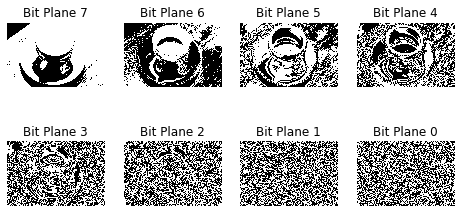## 数字图像位平面分解#

$$f_{k}^{n}{(x,y)} = f_{k}(x,y) {\&} 2^{n} \quad (n = 0, \ldots, 7)$$

## 用 python 来实现数字图像位平面分解#

 1  pip install matplotlib numpy scikit-image 

 1 2 3  import matplotlib.pyplot as plt import numpy as np from skimage import data 

  1 2 3 4 5 6 7 8 9 10  def bitplane(img:np.ndarray): shape = img.shape bits = np.unpackbits(img.reshape(-1, 1), axis=1) plt.figure(figsize=(8,4)) for i in range(8): bit_plane_i = bits[:,i].reshape(shape) plt.subplot(2,4,i+1) plt.imshow(bit_plane_i, cmap="gray") plt.title('Bit Plane {}'.format(7-i)) plt.axis('off') 

 1  image = data.coffee() 
 1  plt.imshow(image);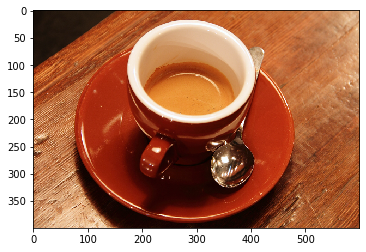1 2 3 4 5 6 7 8 9 10 11 12 13 14 15 16  B = image[:,:,0] G = image[:,:,1] R = image[:,:,2] plt.figure(figsize=(10,8)) plt.subplot(1,3,1) plt.imshow(R, cmap="gray") plt.title('R') plt.subplot(1,3,2) plt.imshow(G, cmap="gray") plt.title('G') plt.subplot(1,3,3) plt.imshow(B, cmap="gray") plt.title('B');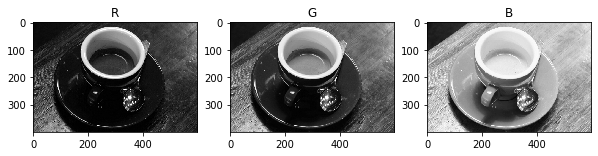1  bitplane(R)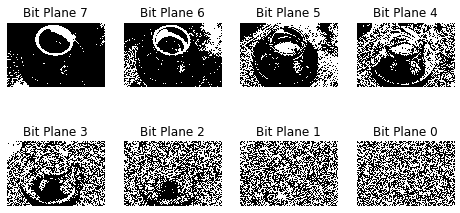1  bitplane(G)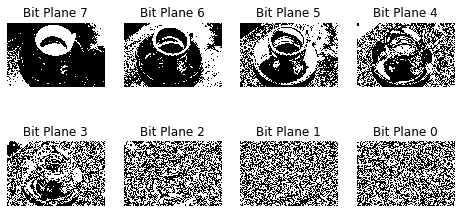1  bitplane(B)